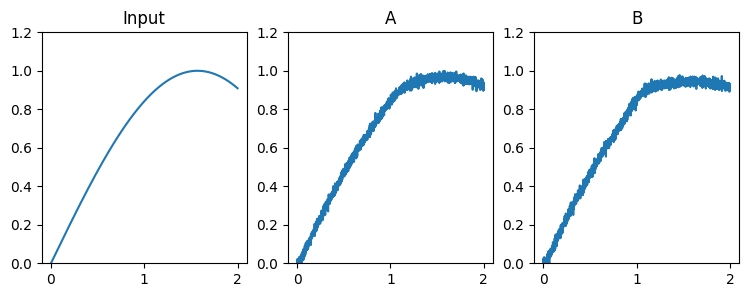Note

This documentation is for a development version. Click here for the latest stable release (v4.0.0).

# Communication channel¶

This example demonstrates how to create a connection from one neuronal ensemble to another that behaves like a communication channel (that is, it transmits information without changing it).

Network diagram:

[Input] ---> (A) ---> (B)


An abstract input signal is fed into the first neuronal ensemble $$A$$, which then passes it on to another ensemble $$B$$. The result is that spiking activity in ensemble $$B$$ encodes the value from the Input.

:

%matplotlib inline
import matplotlib.pyplot as plt
import numpy as np

import nengo


## Step 1: Create the Network¶

:

# Create a 'model' object to which we can add ensembles, connections, etc.
model = nengo.Network(label="Communications Channel")
with model:
# Create an abstract input signal that oscillates as sin(t)
sin = nengo.Node(np.sin)

# Create the neuronal ensembles
A = nengo.Ensemble(100, dimensions=1)
B = nengo.Ensemble(100, dimensions=1)

# Connect the input to the first neuronal ensemble
nengo.Connection(sin, A)

# Connect the first neuronal ensemble to the second
# (this is the communication channel)
nengo.Connection(A, B)


## Step 2: Add Probes to Collect Data¶

Even this simple model involves many quantities that change over time, such as membrane potentials of individual neurons. Typically there are so many variables in a simulation that it is not practical to store them all. If we want to plot or analyze data from the simulation we have to “probe” the signals of interest.

:

with model:
sin_probe = nengo.Probe(sin)
A_probe = nengo.Probe(A, synapse=0.01)  # ensemble output
B_probe = nengo.Probe(B, synapse=0.01)


## Step 3: Run the Model!¶

:

with nengo.Simulator(model) as sim:
sim.run(2)


## Step 4: Plot the Results¶

:

plt.figure(figsize=(9, 3))
plt.subplot(1, 3, 1)
plt.title("Input")
plt.plot(sim.trange(), sim.data[sin_probe])
plt.ylim(0, 1.2)
plt.subplot(1, 3, 2)
plt.title("A")
plt.plot(sim.trange(), sim.data[A_probe])
plt.ylim(0, 1.2)
plt.subplot(1, 3, 3)
plt.title("B")
plt.plot(sim.trange(), sim.data[B_probe])
plt.ylim(0, 1.2)

:

(0.0, 1.2)These plots show the idealized sinusoidal input, and estimates of the sinusoid that are decoded from the spiking activity of neurons in ensembles A and B.

## Step 5: Using a Different Input Function¶

To drive the neural ensembles with different abstract inputs, it is convenient to use Python’s “Lambda Functions”. For example, try changing the sin = nengo.Node line to the following for higher-frequency input:

sin = nengo.Node(lambda t: np.sin(2*np.pi*t))